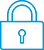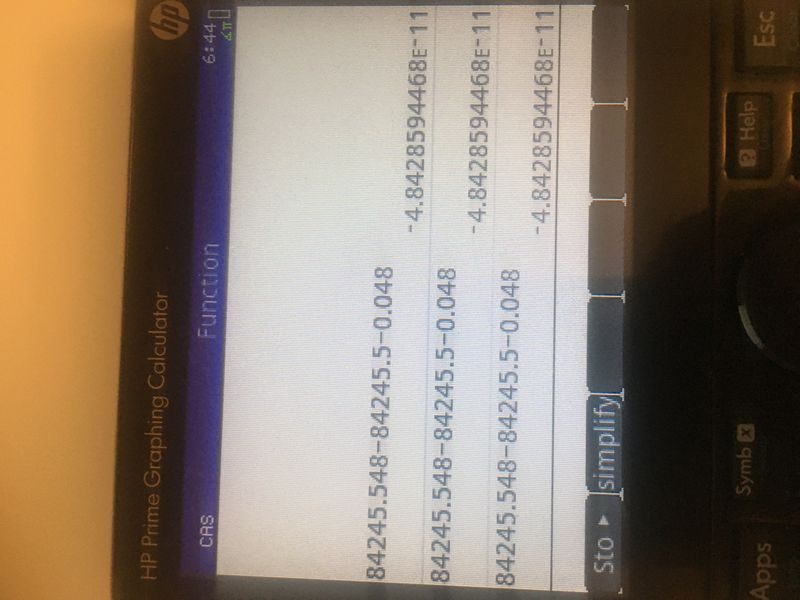cancel
Showing results for
Did you mean:It has been a while since anyone has replied. Simply ask a new question if you would like to start the discussion again.New member
2 1 0 0
Message 1 of 3
708
Flag Post

Solved!

# Wrong result evaluation in CAS mode of HP PrimeHP Recommended
HP Prime

I forgot to turn off the CAS mode when calculating on some chart, but found it returned some weird results which doesn't make sense.Without CAS mode enabled, this would not occur. As the result should be 0 in this case.

No matter how I change the settings in CAS mode, it would not output a correct result.

It makes me worried about my previous calculations. Is this a BUG? The software version of my calculator is 2.1.14433(2020 01 21) with hardware version D, CAS version 1.5.0.

Tags (1)
1 ACCEPTED SOLUTION

Accepted SolutionsLevel 8
641 628 121 214
Message 2 of 3
Flag PostHP Recommended

No, it's not a bug, but is due to the way that CAS uses binary floating point numbers instead of BCD like Home does.  A simple example of this unexpected but understandable behavior is this:

0.5-0.3-0.2 --> 1.7763568394E-15

This does make sense if you keep in mind that CAS represents floating point numbers in binary (not in decimal) using 48 bits for the mantissa, and although 0.5 can be represented exactly in binary, 0.3 and 0.2 cannot, which leads to inevitable roundoff errors.  Same with your example; none of the three numbers in your example can be represented exactly in binary, so CAS uses the best it can do using 48 bits.  The format command helps reveal what's going on:

format(0.5-0.3-0.2, "a12") --> "0x1.0000000000p-49"

format(n, "a12") means "Show me the internal binary representation of n".  The above example means "0.5-0.3-0.2 in CAS gives a result which is exactly equal to 2^(-49)".  So that's the smallest possible error for approximated inputs like these, so CAS actually did the best it could.

In general, use Home for crunching floating-point reals, since it's optimized for that.  Use CAS for exact inputs (that is, numbers which contain no decimal point anywhere) and/or symbolic math, since it's optimized for those operations.  Notice that CAS gets your example exactly correct if the numbers are typed in exact form:

84245548/1000 - 842455/10 - 48/1000 --> 0

People often recommend using the exact() function in CAS to convert floating point reals into exact ratios of integers, but I try to avoid that, since the output of exact() is only an approximation of the input, and so it too can introduce unexpected roundoff errors into a calculation.  Just avoid typing decimal points in CAS and you'll get exact answers.

Hope that helps!  Disclaimer: I don't work for HP. I'm just another happy HP calculator user.

-Joe-
Tags (1)
2 REPLIES 2Level 8
641 628 121 214
Message 2 of 3
Flag PostHP Recommended

No, it's not a bug, but is due to the way that CAS uses binary floating point numbers instead of BCD like Home does.  A simple example of this unexpected but understandable behavior is this:

0.5-0.3-0.2 --> 1.7763568394E-15

This does make sense if you keep in mind that CAS represents floating point numbers in binary (not in decimal) using 48 bits for the mantissa, and although 0.5 can be represented exactly in binary, 0.3 and 0.2 cannot, which leads to inevitable roundoff errors.  Same with your example; none of the three numbers in your example can be represented exactly in binary, so CAS uses the best it can do using 48 bits.  The format command helps reveal what's going on:

format(0.5-0.3-0.2, "a12") --> "0x1.0000000000p-49"

format(n, "a12") means "Show me the internal binary representation of n".  The above example means "0.5-0.3-0.2 in CAS gives a result which is exactly equal to 2^(-49)".  So that's the smallest possible error for approximated inputs like these, so CAS actually did the best it could.

In general, use Home for crunching floating-point reals, since it's optimized for that.  Use CAS for exact inputs (that is, numbers which contain no decimal point anywhere) and/or symbolic math, since it's optimized for those operations.  Notice that CAS gets your example exactly correct if the numbers are typed in exact form:

84245548/1000 - 842455/10 - 48/1000 --> 0

People often recommend using the exact() function in CAS to convert floating point reals into exact ratios of integers, but I try to avoid that, since the output of exact() is only an approximation of the input, and so it too can introduce unexpected roundoff errors into a calculation.  Just avoid typing decimal points in CAS and you'll get exact answers.

Hope that helps!  Disclaimer: I don't work for HP. I'm just another happy HP calculator user.

-Joe-
Tags (1)New member
2 1 0 0
Message 3 of 3
Flag PostHP Recommended